# Hashing by Richard P Simpson Hashing is the

• Slides: 22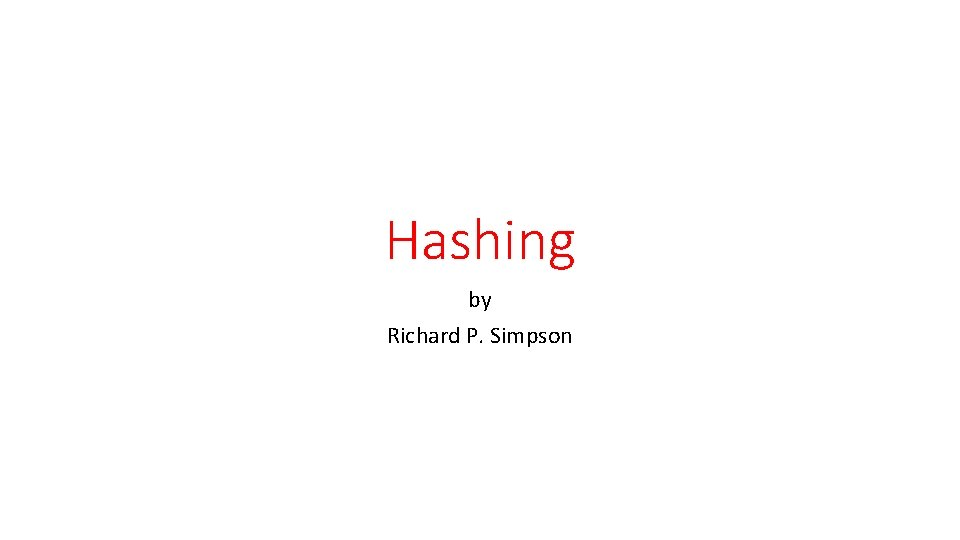Hashing by Richard P. SimpsonHashing is the fastest search method we have In this technique we store items in an array by converting the items key to an integer via a Hash(key) function. This integer is then used to find a position in the array to place the item. For the time being lets assume that the key is an integer and the hash function is very simple i. e. H(key) = key % (tablesize) There are two general schemes that are used • External addressing • Open addressing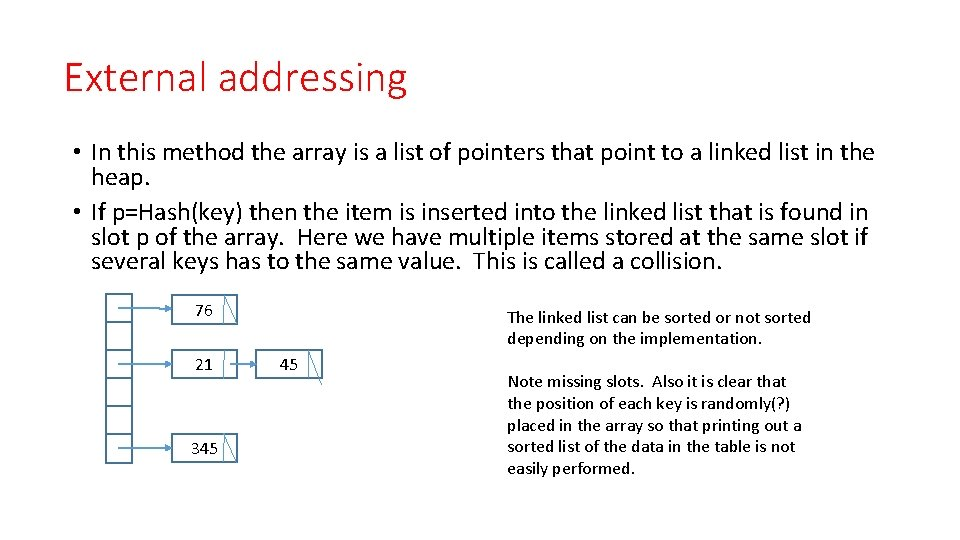External addressing • In this method the array is a list of pointers that point to a linked list in the heap. • If p=Hash(key) then the item is inserted into the linked list that is found in slot p of the array. Here we have multiple items stored at the same slot if several keys has to the same value. This is called a collision. 76 21 345 The linked list can be sorted or not sorted depending on the implementation. 45 Note missing slots. Also it is clear that the position of each key is randomly(? ) placed in the array so that printing out a sorted list of the data in the table is not easily performed.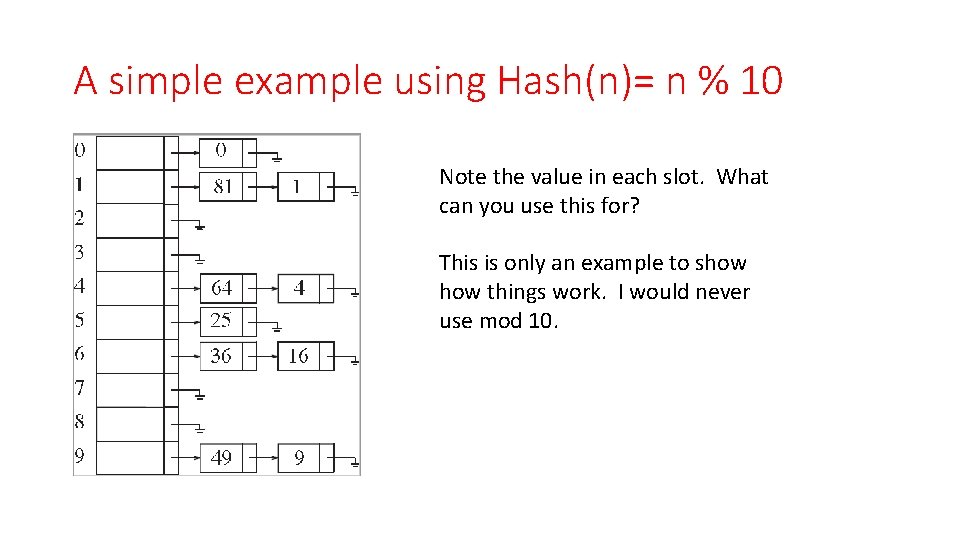A simple example using Hash(n)= n % 10 Note the value in each slot. What can you use this for? This is only an example to show things work. I would never use mod 10.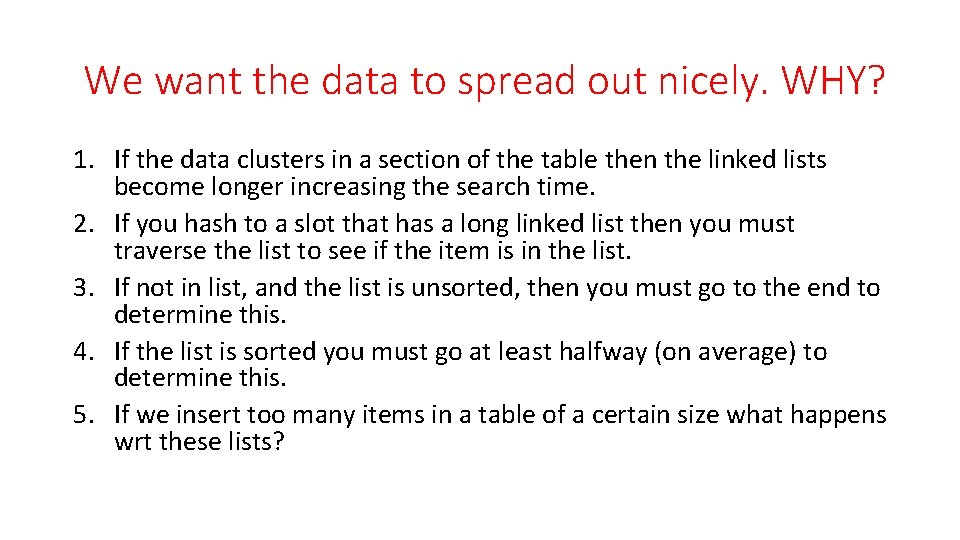We want the data to spread out nicely. WHY? 1. If the data clusters in a section of the table then the linked lists become longer increasing the search time. 2. If you hash to a slot that has a long linked list then you must traverse the list to see if the item is in the list. 3. If not in list, and the list is unsorted, then you must go to the end to determine this. 4. If the list is sorted you must go at least halfway (on average) to determine this. 5. If we insert too many items in a table of a certain size what happens wrt these lists?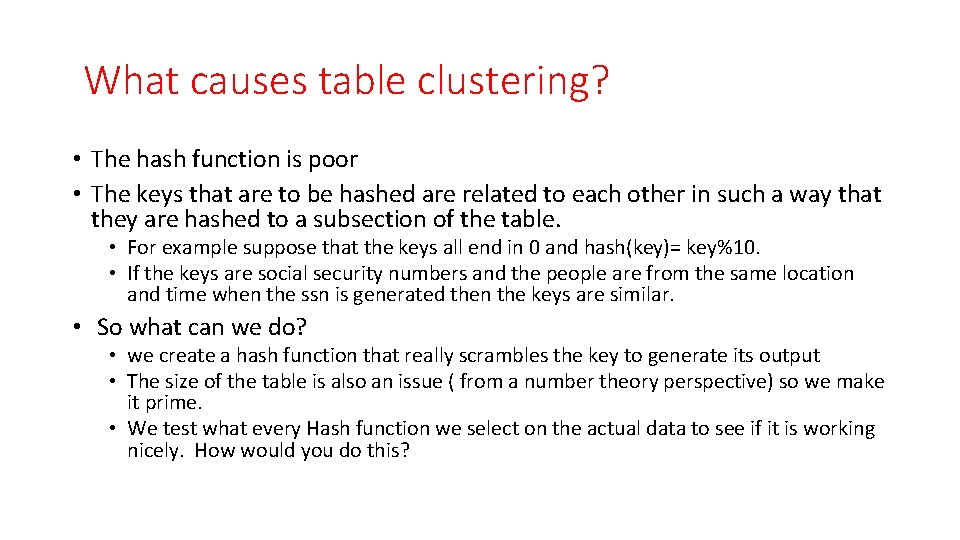What causes table clustering? • The hash function is poor • The keys that are to be hashed are related to each other in such a way that they are hashed to a subsection of the table. • For example suppose that the keys all end in 0 and hash(key)= key%10. • If the keys are social security numbers and the people are from the same location and time when the ssn is generated then the keys are similar. • So what can we do? • we create a hash function that really scrambles the key to generate its output • The size of the table is also an issue ( from a number theory perspective) so we make it prime. • We test what every Hash function we select on the actual data to see if it is working nicely. How would you do this?Hash functions on strings Suppose that the keys are of the form firstname_lastname. Let s=“Richard_Simpson” • first method • Suppose we add up the ascii codes for this string and then mod by the table size. • Why is not a good idea? • A better method. Use Horner’s rule with an appropriate choice of base. • slot = (…((((asc(R) * B + asc(i))*B + asc(c))*B + asc(h))* B + asc(a))* B + …. . ) • slot = slot % tablesize. Is there a problem here? What should B be? • How do you fix this?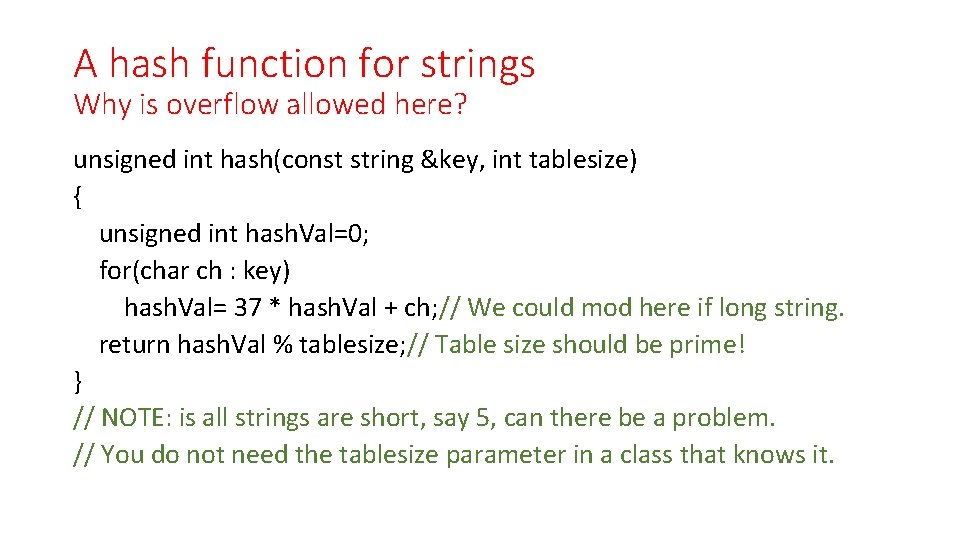A hash function for strings Why is overflow allowed here? unsigned int hash(const string &key, int tablesize) { unsigned int hash. Val=0; for(char ch : key) hash. Val= 37 * hash. Val + ch; // We could mod here if long string. return hash. Val % tablesize; // Table size should be prime! } // NOTE: is all strings are short, say 5, can there be a problem. // You do not need the tablesize parameter in a class that knows it.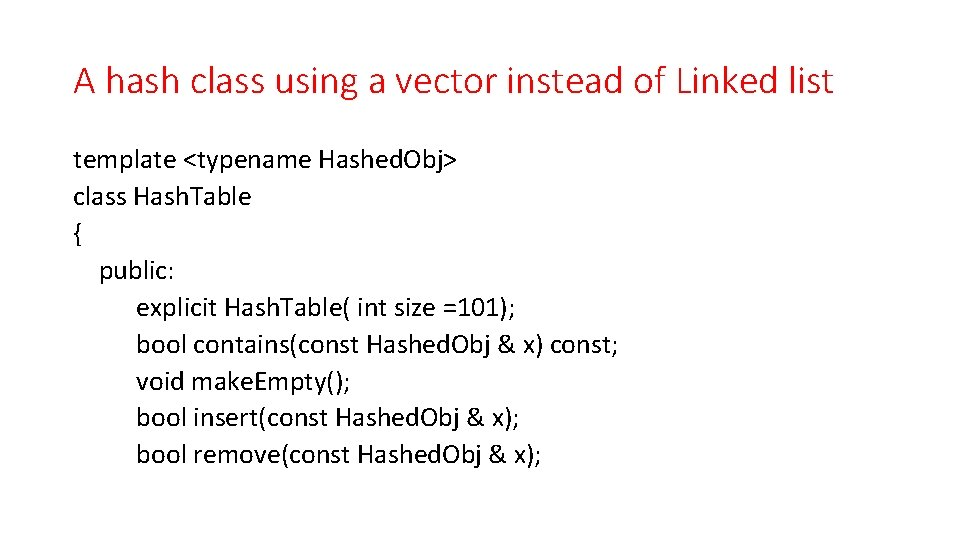A hash class using a vector instead of Linked list template <typename Hashed. Obj> class Hash. Table { public: explicit Hash. Table( int size =101); bool contains(const Hashed. Obj & x) const; void make. Empty(); bool insert(const Hashed. Obj & x); bool remove(const Hashed. Obj & x);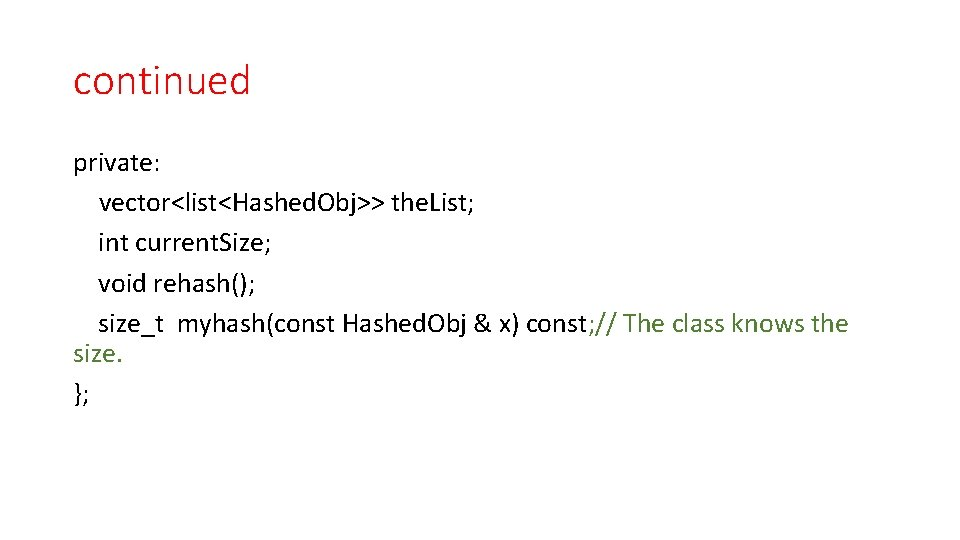continued private: vector<list<Hashed. Obj>> the. List; int current. Size; void rehash(); size_t myhash(const Hashed. Obj & x) const; // The class knows the size. };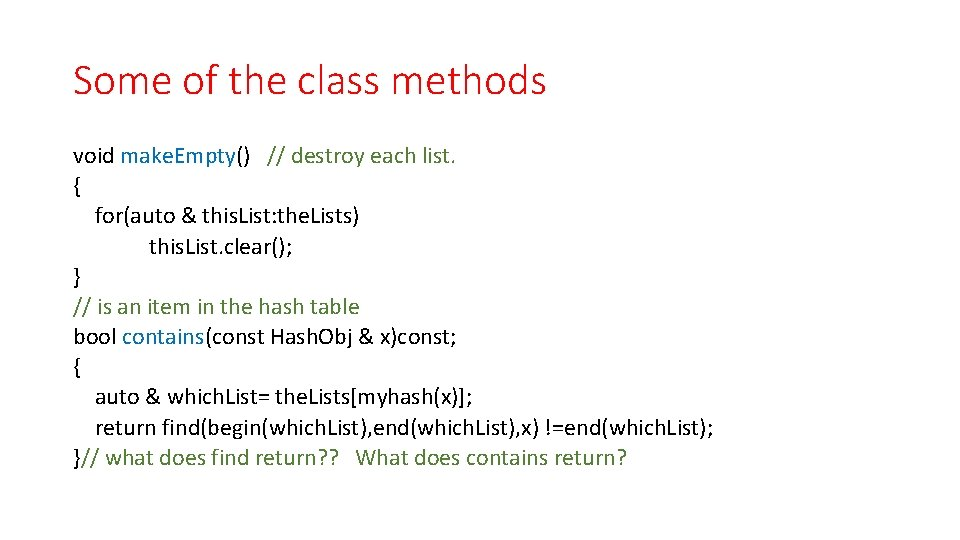Some of the class methods void make. Empty() // destroy each list. { for(auto & this. List: the. Lists) this. List. clear(); } // is an item in the hash table bool contains(const Hash. Obj & x)const; { auto & which. List= the. Lists[myhash(x)]; return find(begin(which. List), end(which. List), x) !=end(which. List); }// what does find return? ? What does contains return?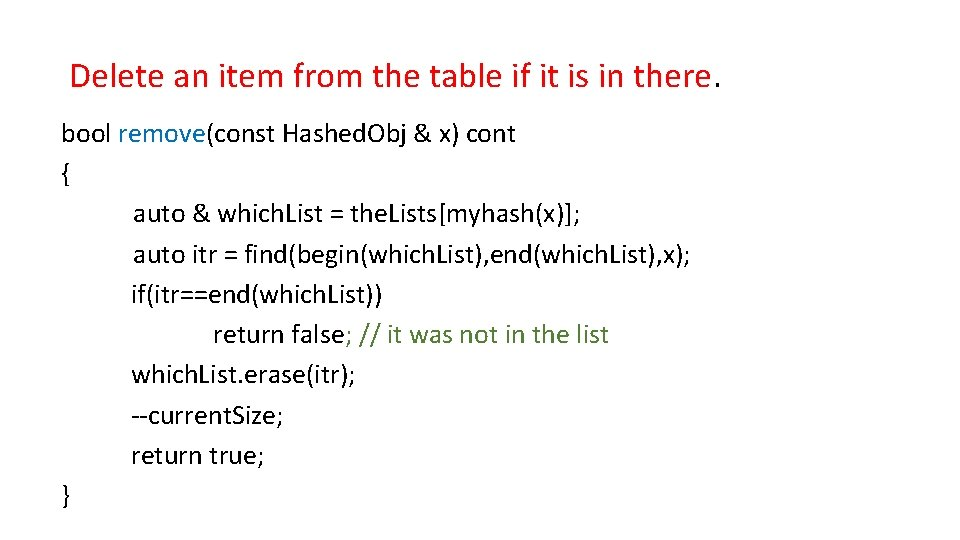Delete an item from the table if it is in there. bool remove(const Hashed. Obj & x) cont { auto & which. List = the. Lists[myhash(x)]; auto itr = find(begin(which. List), end(which. List), x); if(itr==end(which. List)) return false; // it was not in the list which. List. erase(itr); --current. Size; return true; }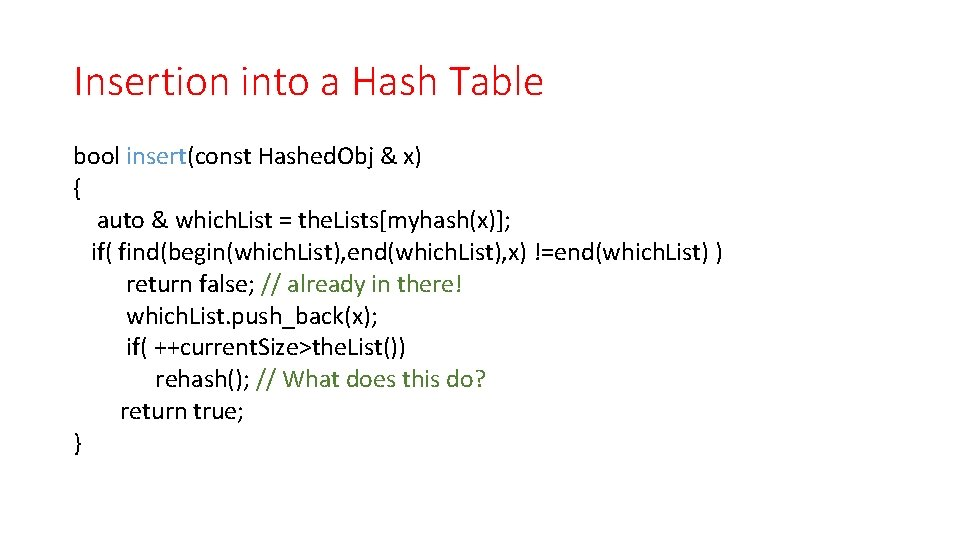Insertion into a Hash Table bool insert(const Hashed. Obj & x) { auto & which. List = the. Lists[myhash(x)]; if( find(begin(which. List), end(which. List), x) !=end(which. List) ) return false; // already in there! which. List. push_back(x); if( ++current. Size>the. List()) rehash(); // What does this do? return true; }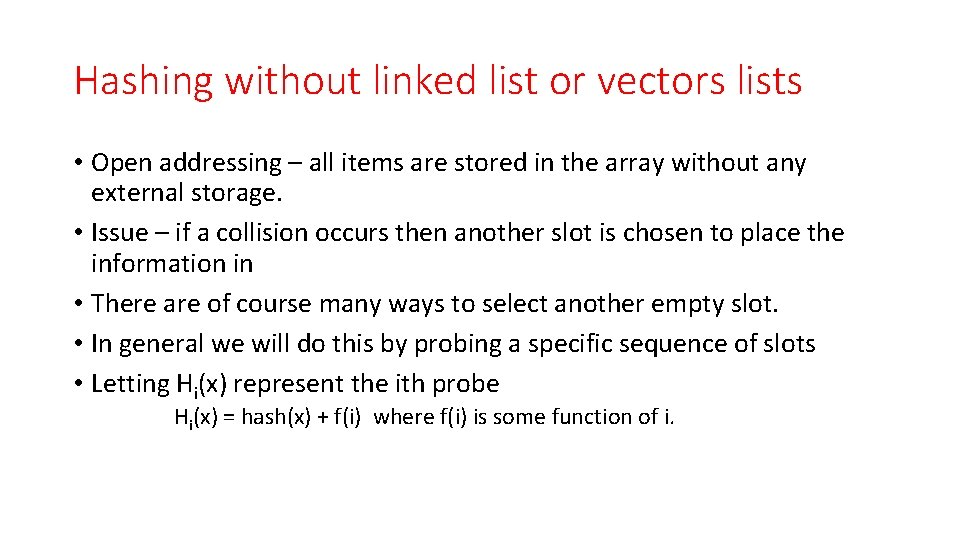Hashing without linked list or vectors lists • Open addressing – all items are stored in the array without any external storage. • Issue – if a collision occurs then another slot is chosen to place the information in • There are of course many ways to select another empty slot. • In general we will do this by probing a specific sequence of slots • Letting Hi(x) represent the ith probe Hi(x) = hash(x) + f(i) where f(i) is some function of i.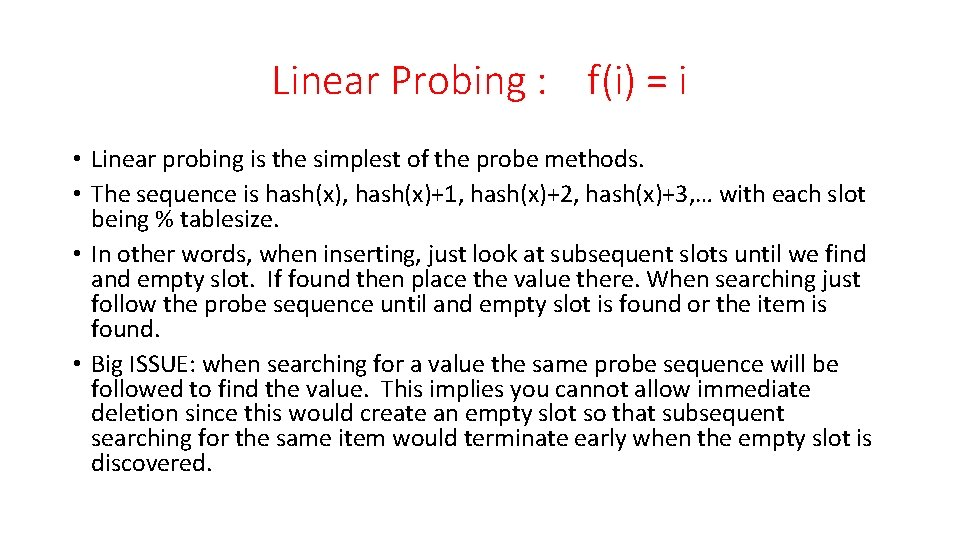Linear Probing : f(i) = i • Linear probing is the simplest of the probe methods. • The sequence is hash(x), hash(x)+1, hash(x)+2, hash(x)+3, … with each slot being % tablesize. • In other words, when inserting, just look at subsequent slots until we find and empty slot. If found then place the value there. When searching just follow the probe sequence until and empty slot is found or the item is found. • Big ISSUE: when searching for a value the same probe sequence will be followed to find the value. This implies you cannot allow immediate deletion since this would create an empty slot so that subsequent searching for the same item would terminate early when the empty slot is discovered.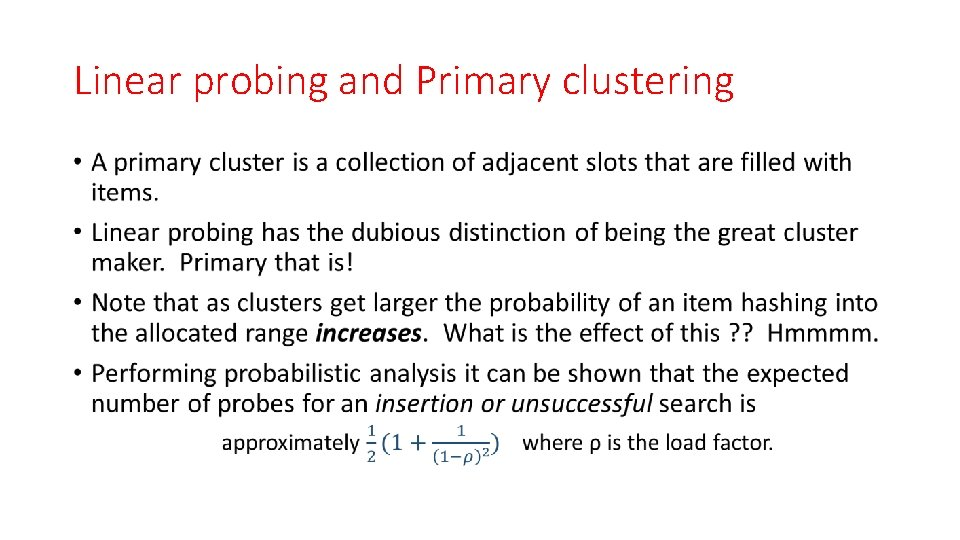Linear probing and Primary clustering •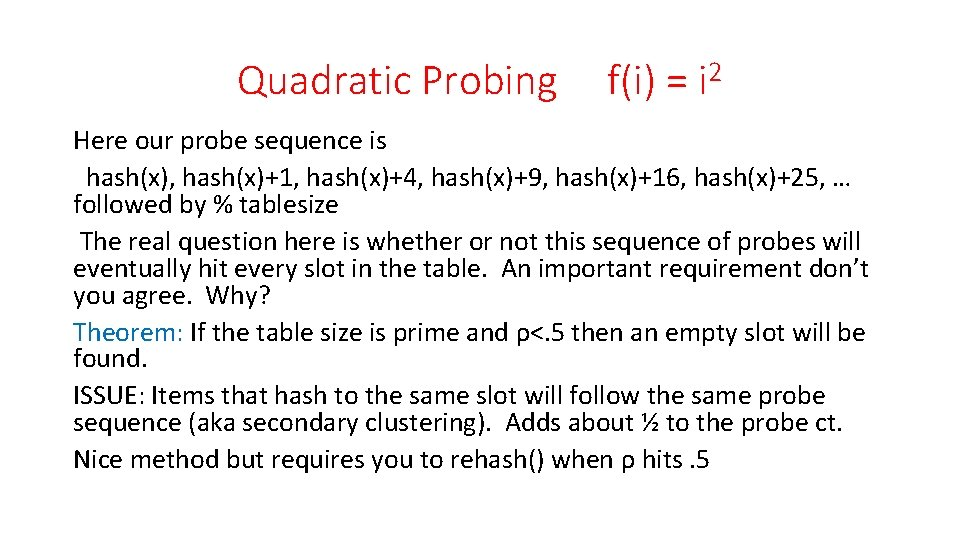Quadratic Probing f(i) = i 2 Here our probe sequence is hash(x), hash(x)+1, hash(x)+4, hash(x)+9, hash(x)+16, hash(x)+25, … followed by % tablesize The real question here is whether or not this sequence of probes will eventually hit every slot in the table. An important requirement don’t you agree. Why? Theorem: If the table size is prime and ρ<. 5 then an empty slot will be found. ISSUE: Items that hash to the same slot will follow the same probe sequence (aka secondary clustering). Adds about ½ to the probe ct. Nice method but requires you to rehash() when ρ hits. 5Double Hashing In this approach we use a second hash function to define the a hop count. This is how far you hop to get to the next slot to check. Hi(x) = hash(x) + i *hash 2(x) The use of the second hash function has the effect of generating a different hop count for every item that hashes to the same slot. Although hash 2(x) effectively kills the possibility of secondary clustering it makes this method more expensive than the previous approaches. You must define hash 2(x) carefully though. 1. Clearly hash 2(x) better not be 0! 2. all the slots must eventually get probed. (Prime table size solves this!)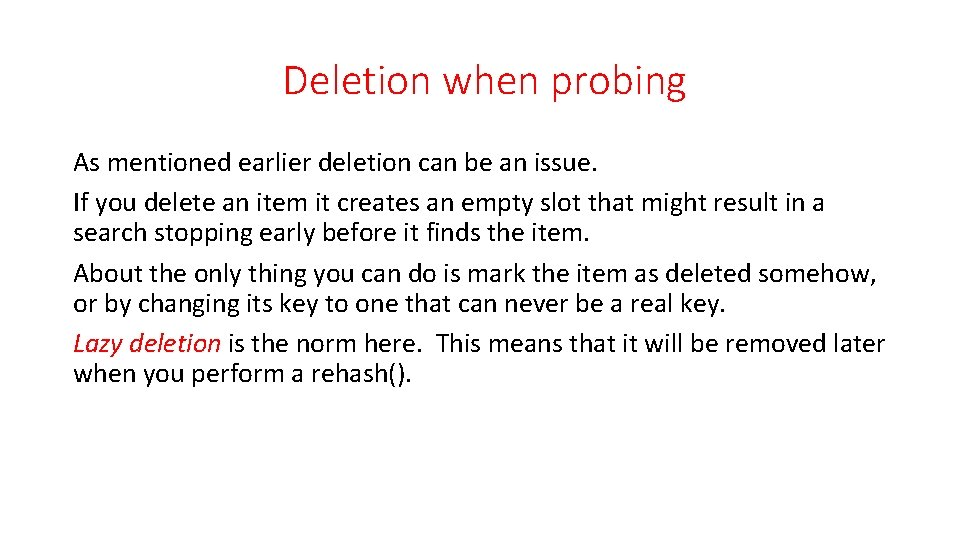Deletion when probing As mentioned earlier deletion can be an issue. If you delete an item it creates an empty slot that might result in a search stopping early before it finds the item. About the only thing you can do is mark the item as deleted somehow, or by changing its key to one that can never be a real key. Lazy deletion is the norm here. This means that it will be removed later when you perform a rehash().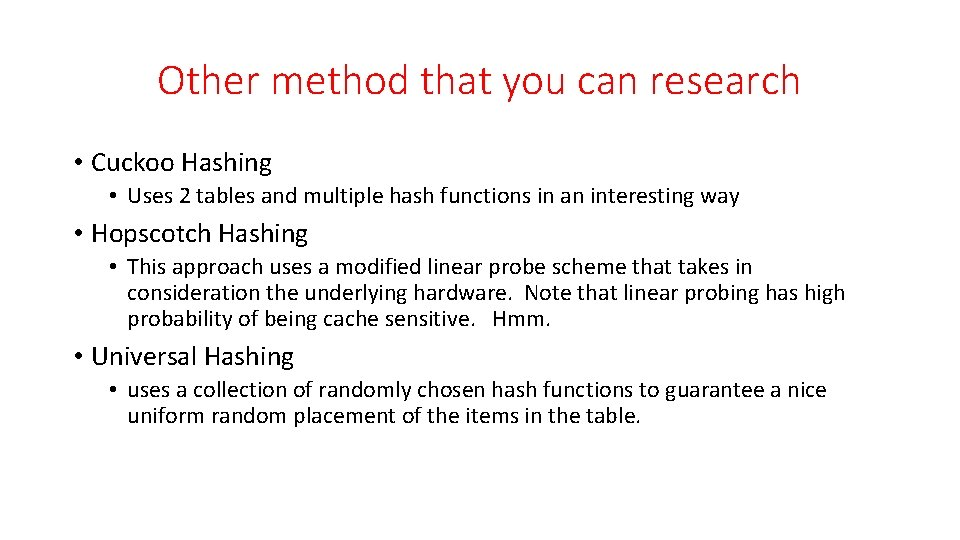Other method that you can research • Cuckoo Hashing • Uses 2 tables and multiple hash functions in an interesting way • Hopscotch Hashing • This approach uses a modified linear probe scheme that takes in consideration the underlying hardware. Note that linear probing has high probability of being cache sensitive. Hmm. • Universal Hashing • uses a collection of randomly chosen hash functions to guarantee a nice uniform random placement of the items in the table.